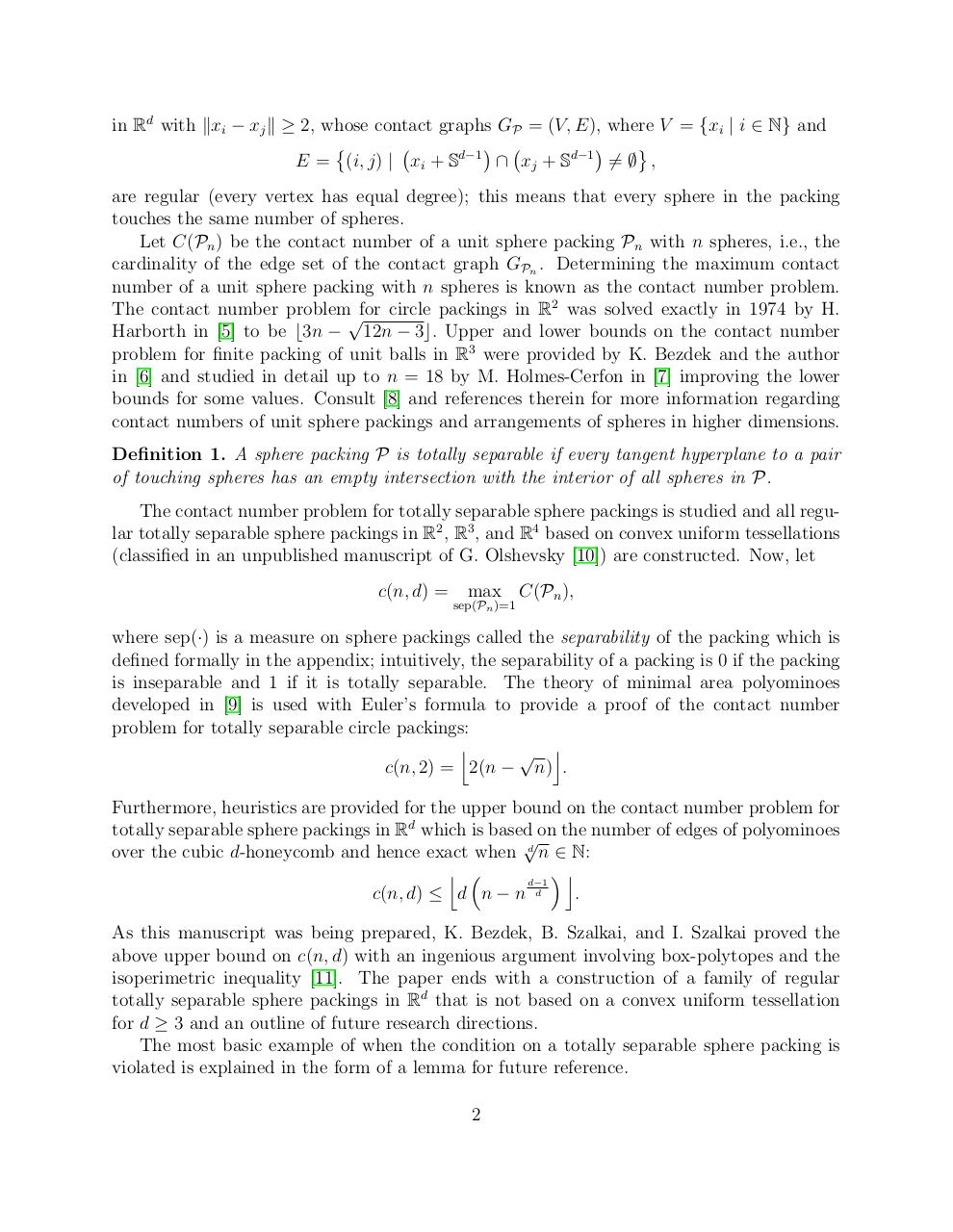# Regular Totally Separable Sphere Packings arXiv.pdfPage 1 2 3 4 5 6 7 8 9 10

#### Text preview

in Rd with kxi − xj k ≥ 2, whose contact graphs GP = (V, E), where V = {xi | i ∈ N} and




E = (i, j) | xi + Sd−1 ∩ xj + Sd−1 6= ∅ ,
are regular (every vertex has equal degree); this means that every sphere in the packing
touches the same number of spheres.
Let C(Pn ) be the contact number of a unit sphere packing Pn with n spheres, i.e., the
cardinality of the edge set of the contact graph GPn . Determining the maximum contact
number of a unit sphere packing with n spheres is known as the contact number problem.
The contact number problem√for circle packings in R2 was solved exactly in 1974 by H.
Harborth in  to be b3n − 12n − 3c. Upper and lower bounds on the contact number
problem for finite packing of unit balls in R3 were provided by K. Bezdek and the author
in  and studied in detail up to n = 18 by M. Holmes-Cerfon in  improving the lower
bounds for some values. Consult  and references therein for more information regarding
contact numbers of unit sphere packings and arrangements of spheres in higher dimensions.
Definition 1. A sphere packing P is totally separable if every tangent hyperplane to a pair
of touching spheres has an empty intersection with the interior of all spheres in P.
The contact number problem for totally separable sphere packings is studied and all regular totally separable sphere packings in R2 , R3 , and R4 based on convex uniform tessellations
(classified in an unpublished manuscript of G. Olshevsky ) are constructed. Now, let
c(n, d) =

max C(Pn ),

sep(Pn )=1

where sep(·) is a measure on sphere packings called the separability of the packing which is
defined formally in the appendix; intuitively, the separability of a packing is 0 if the packing
is inseparable and 1 if it is totally separable. The theory of minimal area polyominoes
developed in  is used with Euler’s formula to provide a proof of the contact number
problem for totally separable circle packings:
j
√ k
c(n, 2) = 2(n − n) .
Furthermore, heuristics are provided for the upper bound on the contact number problem for
totally separable sphere packings in Rd which is based
√on the number of edges of polyominoes
over the cubic d-honeycomb and hence exact when d n ∈ N:
j 
k
d−1
c(n, d) ≤ d n − n d
.
As this manuscript was being prepared, K. Bezdek, B. Szalkai, and I. Szalkai proved the
above upper bound on c(n, d) with an ingenious argument involving box-polytopes and the
isoperimetric inequality . The paper ends with a construction of a family of regular
totally separable sphere packings in Rd that is not based on a convex uniform tessellation
for d ≥ 3 and an outline of future research directions.
The most basic example of when the condition on a totally separable sphere packing is
violated is explained in the form of a lemma for future reference.
2